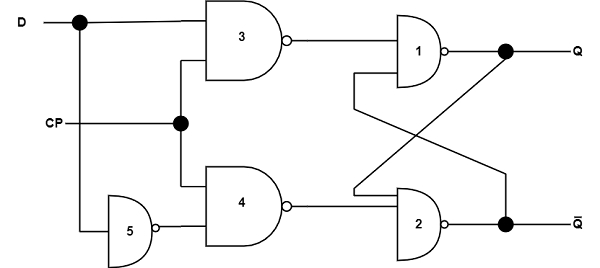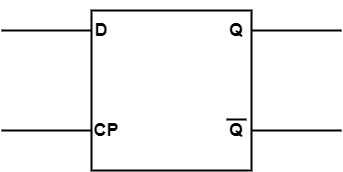# What is D Flip Flop?

The D flip-flop is a clocked flip-flop with a single digital input ‘D’. Each time a D flip-flop is clocked, its output follows the state of ‘D’. The D Flip Flop has only two inputs D and CP. The D inputs go precisely to the S input and its complement is used to the R input.

Considering the pulse input is at 0, the outputs of gates 3 and 4 are at the 1 level and the circuit cannot convert state regardless of the value of D. The D input is sampled when CP = 1. If D is 1, the Q output goes to 1, locating the circuit in the set state. If D is 0, output Q goes to 0, and the circuit switches to a clear state.The truth table for D flip-flop is as shown in the table.

SDQN+1
000
011
100
111

The logic symbol for the D flip-flop is shown in the figure.The D flip flop obtains the destination from its capacity to manage data into its internal storage. This type of flip-flop is known as a gated D-latch. The CP input is provided given the destination G (for gate) to denote that this input allows the gated latch to create applicable data entry into the circuit.

The binary data present at the data input of the D flip flop is changed to the Q output when the CP input is allowed. The output follows the data input considering the pulse continues in its 1 state. When the pulse goes to 0, the binary data that was displayed at the data input at the time the pulse transition appeared is retained at the Q output until the pulse input is allowed again.

The truth table for the D flip flop is displayed in the table. It demonstrates that the next state of the flip flop is independent of the current state since QN+1 is similar to input D whether Q is similar to 0 or 1. This defines that an input pulse will change the value of input D into the output of the flip flop independent of the value of the output earlier the pulse was used.